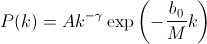This is a test page of the random-group formation. Please enter the following parameters.
• M: total number of elements
• N: total number of groups
• kmax: the number of elements in the largest group
• k0: the number of elements in the smallest group in your data set
Here are a few examples.
• Ulysses by J. Joyce:
• Total number of words M = 263937
• Total number of distinct words N = 29759
• The most common word ('the') appears kmax = 14924 times.
• There are many words used only once throughout the novel: k0 = 1.
• Collected works by H. Melville:
• Total number of words M = 743666
• Total number of distinct words N = 30122
• The most common word ('the') appears kmax = 49136 times.
• There are many words used only once throughout the works: k0 = 1.

The resulting numbers mean that the frequency distribution is predicted to beand kc does not explicitly appear in this expression.

Warning: it may take some time.
Please be patient and use the reload button to stop or go to another calculation.